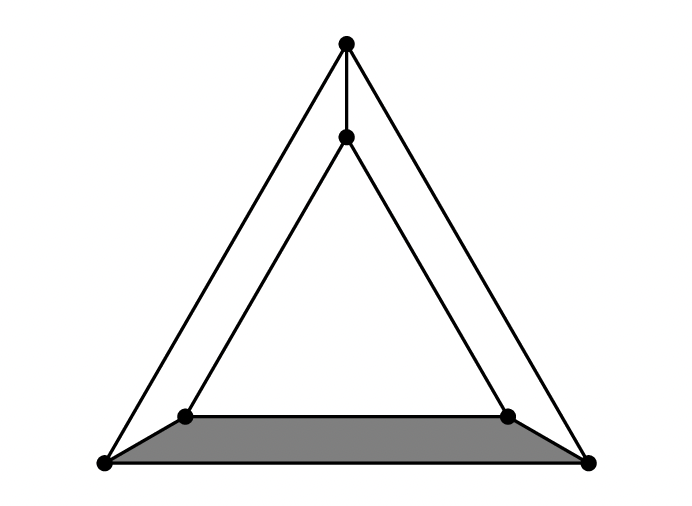## AMC 8 Question

An equilateral triangle is placed inside a larger equilateral triangle so that the region between them can be divided into three congruent trapezoids, as shown below. The side length of the inner triangle is : 6 the side length of the larger triangle. What is the ratio of the area of one trapezoid to the area of the inner triangle?(A) (B) (C) (D) Answer: (A) (B) (C) (D)